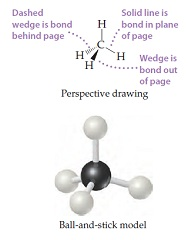# Problem: The methane molecule, CH4, has the geometry shown in following figure. Imagine a hypothetical process in which the methane molecule is "expanded," by simultaneously extending all four C–H bonds to infinity. We then have the process CH4(g) → C(g) + 4 H(g).Compare this process with the reverse of the reaction that represents the standard enthalpy of formation of CH4(g). Which is the more endothermic process?

###### FREE Expert Solution

CH4(g) → C(g) + 4 H(g).

• involves one compound forming two species→ bond breaking
endothermic

81% (107 ratings)###### Problem Details
The methane molecule, CH4, has the geometry shown in following figure. Imagine a hypothetical process in which the methane molecule is "expanded," by simultaneously extending all four C–H bonds to infinity. We then have the process CH4(g) → C(g) + 4 H(g).Compare this process with the reverse of the reaction that represents the standard enthalpy of formation of CH4(g). Which is the more endothermic process?

Frequently Asked Questions

What scientific concept do you need to know in order to solve this problem?

Our tutors have indicated that to solve this problem you will need to apply the Endothermic & Exothermic Reactions concept. You can view video lessons to learn Endothermic & Exothermic Reactions. Or if you need more Endothermic & Exothermic Reactions practice, you can also practice Endothermic & Exothermic Reactions practice problems.